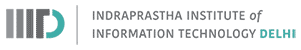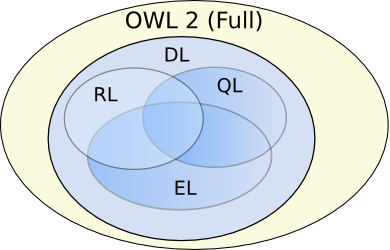## Ontology Reasoning

Monsoon 2018
Instructor: Raghava Mutharaju
IIIT-Delhi### Ontology Reasoning

• Reasoning is the process of deriving facts that are not explicitly expressed in the ontology
• Reasoning makes implicit facts explicit
• Logical consequences are drawn from the axioms in the ontology

### Ontology Reasoning

• Axioms before reasoning
• {john} $\sqsubseteq$ USCitizen
• USCitizen $\sqsubseteq \exists$hasPassport.USPassport
• $\exists$hasPassport.USPassport $\sqsubseteq$ EUVisaNotRequired
• Logical consequences after reasoning
• USCitizen $\sqsubseteq$ EUVisaNotRequired
• {john} $\sqsubseteq$ EUVisaNotRequired

### Purpose of Ontology Reasoning

1. Design and maintain high quality ontologies
• Consistency: reasoner helps in determining whether an ontology is consistent or not
• Minimally redundant: reasoner checks for unintended synonyms
2. Answer queries over ontology classes and instances
• Find more general/specific classes
• Retrieve individuals of a class

### Expressivity and Complexity Trade-off

#### OWL 2 ProfilesImage source: https://goo.gl/HDvOZe

#### Reasoning Complexity

• OWL 2 Full: Undecidable
• OWL 2 DL: N2EXPTIME (22n in the size of input)
• OWL 2 EL, OWL 2 RL, OWL 2 QL: Polynomial time

### Types of Reasoning

• Forward chaining
• Start with the axioms and apply inference rules repeatedly until no more new axioms can be derived
• Saves time during querying but ends up using more space
• Backward chaining
• Start with a query and apply inference rules that are needed to only answer the query
• Saves space but increases the querying time

### Important Reasoning Tasks

• Consistency of the knowledge base (ontology in our case)
• Class level consistency (is class C empty, $C \equiv \bot$)
• Class inclusion (is class C a subclass of class D)
• Class equivalence
• Class disjointness
• Class membership (does an instance a belong to class C?)
• Instance retrieval (list all the instances of class C)

### Reasoning Procedure

• Rule based reasoning
• Tableaux algorithm

### Rule based reasoning

• If $X \sqsubseteq A$ and $A \sqsubseteq B$ then $X \sqsubseteq B$
• If $X \sqsubseteq A_1$ and $X \sqsubseteq A_2$ then $X \sqsubseteq A_1 \sqcap A_2$
• If $A \sqsubseteq \exists r.B$ and $\exists r.B \sqsubseteq C$, then $A \sqsubseteq C$
• If $A \sqsubseteq \exists r.B$, $B \sqsubseteq C$ and $\exists r.C \sqsubseteq D$, then $A \sqsubseteq D$
• If $A \sqsubseteq \exists r.B$, $r \sqsubseteq s$ and $\exists s.B \sqsubseteq C$, then $A \sqsubseteq C$
• If $A \sqsubseteq \exists r.B$, $r \sqsubseteq s$, $B \sqsubseteq C$ and $\exists s.C \sqsubseteq D$, then $A \sqsubseteq D$

### Tableaux Algorithm

• They are used to test satisfiability (consistency)
• A tree is built with nodes consisting of class expressions to be evaluated
• Edges are labelled with the relationships between classes
• Nodes are expanded by applying the tableaux rules
• Tree expansion stops when either no more rules can be applied or a clash occurs (for eg: $A \sqcap \neg A$)

## References

1. OWL 2 Profiles. Boris Motik et. al. 2012